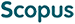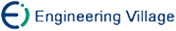Li Panchi, Zhou Hongyan. Model and Algorithm of Quantum Neural Network Based on the Controlled Hadamard Gates[J]. Journal of Computer Research and Development, 2015, 52(1): 211-220. doi: 10.7544/issn1000-1239.2015.20131016
 Citation: Li Panchi, Zhou Hongyan. Model and Algorithm of Quantum Neural Network Based on the Controlled Hadamard Gates[J]. Journal of Computer Research and Development, 2015, 52(1): 211-220. doi: 10.7544/issn1000-1239.2015.20131016# Model and Algorithm of Quantum Neural Network Based on the Controlled Hadamard Gates• To enhance the approximation capability of neural network, a quantum neural network model based on the controlled-Hadamard gates is proposed. This model takes a multi-dimensional discrete sequence as the input, which can be described by a matrix where the number of rows denotes the number of input nodes, and the number of columns denotes the length of discrete sequence. This model consists of three layers, the hidden layer consists of quantum neurons, and the output layer consists of common neurons. The quantum neuron consists of the quantum rotation gates and the multi-qubits controlled-Hadamard gates. Using the information feedback of target qubit from output to input in multi-qubits controlled-Hadamard gates, the overall memory of input sequence is realized. The output of quantum neuron is obtained from the controlled relationship between the control bits and target bit of controlled-Hadamard gates. The learning algorithm is designed in detail according to the basis principles of quantum computation. The characteristics of input sequence can be effectively obtained. The experimental results show that, when the input nodes and the length of the sequence satisfy certain relations, the proposed model is obviously superior to the common BP neural network.
•/DownLoad:  Full-Size Img  PowerPoint